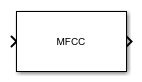# MFCC

Extract mel-frequency cepstral coefficients from audio

Since R2022b

•Libraries:
Audio Toolbox / Features

## Description

The MFCC block extracts feature vectors containing the mel-frequency cepstral coefficients (MFCCs), as well as their delta and delta-delta features, from the audio input signal. MFCCs are popular features extracted from speech signals for use in classification tasks.

## Ports

### Input

expand all

Audio input signal, specified as a column vector or a matrix. When you specify a matrix, the block treats columns as independent audio channels.

Data Types: `single` | `double`

### Output

expand all

MFCC features returned as a matrix or 3-D array. The features include the MFCCs themselves and optionally include the delta and delta-delta features of the MFCCs. The dimensions of the output are L-by-M-by-N, where:

Trailing dimensions of size 1 are removed from the output.

Data Types: `single` | `double`

## Parameters

expand all

Mel-Frequency Cepstral Coefficients

Analysis window applied to the input signal in the time domain, specified as a real vector.

Number of overlapping samples between adjacent windows, specified as an integer in the range [0, `windowLength`), where `windowLength` is the length of the analysis window and is specified by the Window parameter.

Number of cepstral coefficients in each feature vector, specified as a positive integer greater than 1.

Type of nonlinear rectification applied to the spectrum prior to the discrete cosine transform, specified as `Logarithm` or ```Cubic root```.

When you select this parameter, the block appends the delta of the MFCCs to the coefficients in each feature vector. The delta is an approximation of the first derivative of the MFCCs with respect to time. The number of delta features is equal to the number of MFCCs, which is specified by Number of cepstral coefficients.

When you select this parameter, the block appends the delta-delta of the MFCCs to each output feature vector. The delta-delta is an approximation of the second derivative of the MFCCs with respect to time. The number of delta-delta features is equal to the number of MFCCs, which is specified by Number of cepstral coefficients.

The block appends the delta-delta after the delta in the feature vectors if you also select the Append delta parameter.

Number of coefficients for calculating delta and delta-delta, specified as an odd integer greater than 2.

Output Buffering

Number of MFCC feature vectors in output, specified as a positive integer. The block buffers the output to return the specified number of feature vectors.

Number of feature vectors the block overlaps in the output, specified as a nonnegative integer less than Number of feature vectors.

Simulation Parameters

When you select this parameter, the block inherits its sample rate from the input signal. When you clear this parameter, you specify the sample rate in the Input sample rate (Hz) parameter.

Input sample rate in Hz, specified as a positive scalar.

#### Dependencies

To enable this parameter, clear the Inherit sample rate from input parameter.

Mel Filter Bank Design

Number of bands in mel filter bank, specified as a positive integer.

When you select this parameter, the block sets the Frequency range to `[0,fs/2]`, where `fs` is the sample rate. The block determines the sample rate using the Inherit sample rate from input and Input sample rate (Hz) parameters.

Frequency range in Hz of mel filter bank, specified as a two-element row vector.

#### Dependencies

To enable this parameter, clear the Auto-determine frequency range parameter.

Design domain of mel filter bank, specified as `linear` or `warped`.

Normalization technique that the block uses for the filter bank weights, specified as `bandwidth`, `area`, or `none`.

• `bandwidth` –– Normalize the weights of each bandpass filter by the corresponding bandwidth of the filter.

• `area` –– Normalize the weights of each bandpass filter by the corresponding area of the bandpass filter.

• `none` –– The block does not normalize the weights of the filters.

Spectrogram

When you select this parameter, the block applies window normalization.

Type of spectrum, specified as `power` or `magnitude`.

When you select this parameter, the block automatically sets the FFT length to the window length. The window length is determined by the Window parameter.

Number of points used to calculate the DFT, specified as a positive integer.

#### Dependencies

To enable this parameter, clear the Auto-determine FFT length parameter.

## Block Characteristics

 Data Types `double` | `single` Direct Feedthrough `no` Multidimensional Signals `no` Variable-Size Signals `no` Zero-Crossing Detection `no`

expand all

## Version History

Introduced in R2022b

expand all